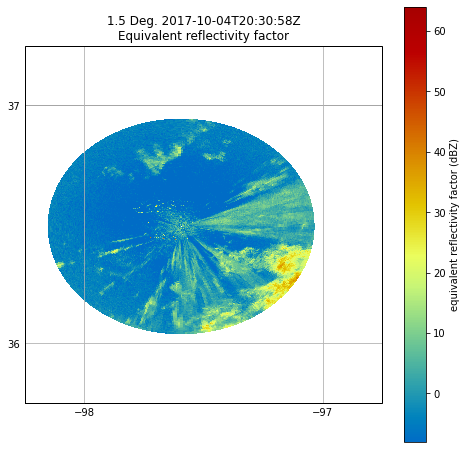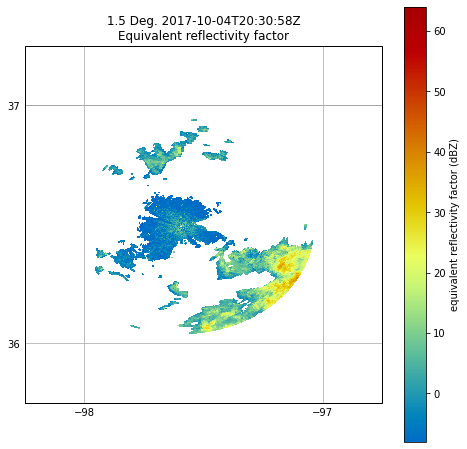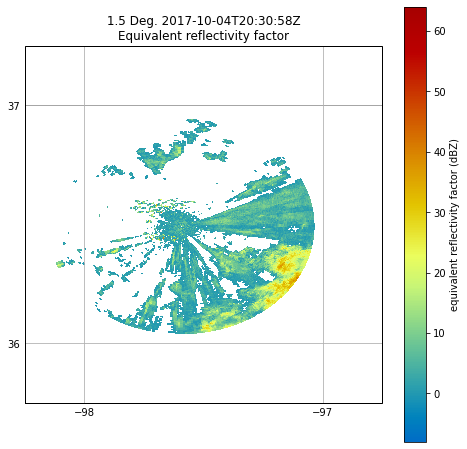# Masking Data With Py-ART Gatefilters#

# This notebook gives examples on how to use a Py-ART GateFilter object to mask data.

# First import needed modules.
import cartopy.crs as ccrs
import matplotlib.pyplot as plt
import numpy as np
import pyart

## You are using the Python ARM Radar Toolkit (Py-ART), an open source
## library for working with weather radar data. Py-ART is partly
## supported by the U.S. Department of Energy as part of the Atmospheric
## Radiation Measurement (ARM) Climate Research Facility, an Office of
## Science user facility.
##
## If you use this software to prepare a publication, please cite:
##
##     JJ Helmus and SM Collis, JORS 2016, doi: 10.5334/jors.119

# Read the radar file.

# See what moments are present.

dict_keys(['total_power', 'reflectivity', 'velocity', 'spectrum_width', 'differential_reflectivity', 'specific_differential_phase', 'cross_correlation_ratio', 'normalized_coherent_power', 'differential_phase', 'xsapr_clutter', 'sounding_temperature', 'height', 'signal_to_noise_ratio', 'velocity_texture', 'gate_id', 'simulated_velocity', 'corrected_velocity', 'unfolded_differential_phase', 'corrected_differential_phase', 'filtered_corrected_differential_phase', 'corrected_specific_diff_phase', 'filtered_corrected_specific_diff_phase', 'corrected_differential_reflectivity', 'corrected_reflectivity', 'height_over_iso0', 'specific_attenuation', 'path_integrated_attenuation', 'specific_differential_attenuation', 'path_integrated_differential_attenuation', 'rain_rate_A'])

# When plotting, we can see second trip echoes present as well as nonhydrometeor echoes.
fig = plt.figure(figsize=[8, 8])
display.plot_ppi_map(
"reflectivity",
sweep=2,
resolution="50m",
vmin=-8,
vmax=64,
min_lon=-98.25,
max_lon=-96.75,
min_lat=35.75,
max_lat=37.25,
projection=ccrs.PlateCarree(),
)
plt.show()# When both displaying and analyzing such data, it is desirable to remove such echoes.
# Py-ART provides the GateFilter class for doing such filtering.

# The below example shows how you can use a gatefilter to exclude values where gate_id is
# equal to a certain value. (You can exclude values based on conditions from multiple
# fields. In this example, we are excluding regions identified as second trip echoes
# (gate_id = 0), nonhydrometeors (gate_id = 1), and clutter (gate_id = 2).
gatefilter.exclude_equal("gate_id", 0)
gatefilter.exclude_equal("gate_id", 3)
gatefilter.exclude_equal("gate_id", 5)

# The gatefilter can be then be specified as an input to plot_ppi_map()
# As you can see, much cleaner with the clutter removed.
fig = plt.figure(figsize=[8, 8])
display.plot_ppi_map(
"reflectivity",
sweep=2,
resolution="50m",
vmin=-8,
vmax=64,
min_lon=-98.25,
max_lon=-96.75,
min_lat=35.75,
max_lat=37.25,
projection=ccrs.PlateCarree(),
gatefilter=gatefilter,
)
plt.show()# Let's reset the gatefilter and try another method.

# Lets remove reflectivity values below a threshold.
gatefilter.exclude_below("reflectivity", 0)

# Here we see reflectivity values below zero masked.
fig = plt.figure(figsize=[8, 8])
display.plot_ppi_map(
"reflectivity",
sweep=2,
resolution="50m",
vmin=-8,
vmax=64,
min_lon=-98.25,
max_lon=-96.75,
min_lat=35.75,
max_lat=37.25,
projection=ccrs.PlateCarree(),
gatefilter=gatefilter,
)
plt.show()# The structure of the gatefilter includes boolean arrays of the same shape as the radar
# fields that tell you whether a gate is excluded (gatefilter.gate_excluded) or included
# (gatefilter.gate_included). These can be used to do calculations on the data that
# include the filter. For example, if we want to get the mean reflectivity of each sweep, we can do:

[0.9344453454202706, -0.620691732853737, -2.3756584054113996, -5.597935968662154, -7.696735014111216, -9.061894569912447, -9.721673265240488, -10.086695918593689, -10.33930700509078, -10.599363169483158, -10.836875745223637, -11.051601529570966, -11.22435547210836, -11.378109469982695, -11.553820234067336, -11.648943841665002, -11.837593740986444, -12.049614721040282, -12.331202567459613, -12.585432697375357, -12.87002341270722, -12.993012637722325, -13.061966001642219, -13.122799449619672]